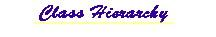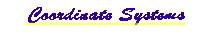## mil.navy.nps.rra Class Beam

```java.lang.Object
|
+--mil.navy.nps.rra.Beam
```

public class Beam
extends java.lang.Object

A Beam is defined as the volume swept out by four Rays as they traverse the ocean environment.

This class forms a Beam from four Ray calculations, which are acoustically propagated according to the sound speed profile (SSP) & Surface & Bottom. Calculated values can be used to produce a VRML representation of the Beam.

Beams of energy can be considered to consist of bundles of sound rays. The energy in a bundle can be shown not to diverge from the bundle. Thus the energy in a bundle is constant, and subsequently the product of the intensity versus the area of the bundle perpendicular to direction of propagation is a constant as well. Therefore a beam is a fundamental building block for constructing a multiple-beam Lobe of a sonar pattern.

Version:
1.0

Author:
LT Timothy M. Holliday USN

Contact: Don Brutzman (web.nps.navy.mil/~brutzman) brutzman@nps.navy.mil

Source Code:
~/vrtp/mil/navy/nps/rra/Beam.java
http://devo.stl.nps.navy.mil/~brutzman/vrtp/mil/navy/nps/rra/Beam.javaHistory:
 15 November 97 Timothy M. Holliday New 17 March 98 Timothy M. Holliday Added HTML comment convention 12 April 98 Timothy M. Holliday Parameterless Constructors 14 April 98 Timothy M. Holliday Simplified VRML Routines 21 April 98 Timothy M. Holliday Fixed Problem with calculateSoundPressureLevel 16 October 98 Don Brutzman Javadoc updates for jdk1.2b4 24 April 99 Don Brutzman Javadoc updates for jdk1.2.1

Reference:
Holliday, Timothy, Real-Time 3D Sonar Modeling and Visualization, Master's Thesis, Naval Postgraduate School, Monterey California, June 1998. Available at vrtp/rra/HollidayRRAthesis.pdf. and devo.stl.nps.navy.mil/~brutzman/vrtp/rra/HollidayRRAthesis.pdf. Slideset available in Powerpoint and Acrobat .pdf formats.

`Ray`, `Lobe`, `Bottom`, `Surface`, `SSP`, `ExampleBeamStatic`, `ExampleBeamDynamic`, `Vec3d`

 Field Summary `static int` `NONE`           Enumeration flag to avoid further attenuation of color intensity. `static int` `T_L`           Enumeration flag to attenuate color intensity by transmission loss (T_L, pronounced "T sub L"). `static int` `TIME`           Enumeration flag to attenuate color intensity by timestep.

 Constructor Summary `Beam()`           Use accessor methods to set initial values of member variables.

 Method Summary ` void` `calculateBeam(Targets targets)`           This method calculates the trajectory of the beam of energy enclosed by the defining rays of the beam tube. ` void` ```calculateSoundPressureLevel(double[][][] field, double deltaRange, double deltaDepth, double frequency)```           This method calculates the trajectory of the beam of energy enclosed by the defining rays of the beam tube. ` java.lang.String` `dynamicVRML()`           This method creates a dynamic VRML string shape that is the three dimensional representation of the beam pulse that is propogated. `static boolean` `getAppendLineFeed()`           This is a static method that returns the current line appendage. ` double` `getAzimuth()`           This method returns the azimuthal angle. ` int` `getBeamNumber()`           This returns BeamNumber. ` Bottom` `getBottom()`           This method returns the handle to the bottom object. ` double` `getDeltaTime()`           This method returns the simulation time step. ` int` `getDetectCount()`           This method returns the total number of detects by the beam ` double` `getDetectEchoLevel(int N)`           This method returns the echo level of the detected target ` double` `getDetectTime(int N)`           This method returns the detect time for a given detect in the beam ` int` `getDuration()`           This method sets the duration of the sonar pulse. ` double` `getElevation()`           This method returns the elevation angle. ` double` `getEndTime()`           This method returns the simulation end time. ` double` `getHalfBeamWidthX()`           This method returns the half beam width of the beam in the azimuthal direction. ` double` `getHalfBeamWidthY()`           This method returns the half beam width of the beam in the azimuthal direction. ` Vec3d` `getPosition()`           This method returns the position of the beam. ` SSP` `getSsp()`           This method returns the handle to the sound speed profile object. ` Surface` `getSurface()`           This method returns the handle to the surface object. ` java.lang.String` `pingTimerVRML()`           This method returns a VRML timer string that contains the appropriate timing information for the beam ` void` `reset()`           This method resets all of the beam parameters after instanciation has occurred, since reuse is more time efficient than garbage collection and reallocation. `static void` `setAppendLineFeed(boolean pAppendLineFeed)`           This is a static method used to indicate whether a line feed is desired at the end of every line. ` void` `setAzimuth(double phi)`           This method sets the azimuthal angle, which is the angle from the x-axis to the z-axis rotating about the y-axis. ` void` `setBeamNumber(int number)`           This method sets BeamNumber. ` void` `setBottom(Bottom pBottom)`           This method sets the handle to the bottom object. ` void` `setDeltaTime(double pDeltaTime)`           This method sets the time step in the simulation. ` void` `setDuration(int duration)`           This method sets the duration of the sonar pulse. ` void` `setElevation(double beta)`           This method sets the elevation angle, which is the angle from the y-axis to the x-axis rotating about the z-axis . ` void` `setEndTime(double pEndTime)`           This method sets the simulation end time. ` void` `setHalfBeamWidthX(double pHalfBeamWidthX)`           This method sets the half beam width of the beam in the azimuthal direction. ` void` `setHalfBeamWidthY(double pHalfBeamWidthY)`           This method sets the half beam width of the beam in the elevation direction. ` void` ```setPosition(double x, double y, double z)```           This method sets the position of the beam. ` void` `setSsp(SSP pSsp)`           This method sets the handle to the sound speed profile object. ` void` `setSurface(Surface pSurface)`           This method sets the handle to the surface object. ` java.lang.String` ```staticVRML(int colorChoice, int intensityChoice)```           This method writes to the console a VRML shape that is the three dimensional representation of the beam that is propagated.

 Methods inherited from class java.lang.Object ```, clone, equals, finalize, getClass, hashCode, notify, notifyAll, toString, wait, wait, wait```

 Field Detail

### T_L

`public static final int T_L`
Enumeration flag to attenuate color intensity by transmission loss (T_L, pronounced "T sub L").

### TIME

`public static final int TIME`
Enumeration flag to attenuate color intensity by timestep.

### NONE

`public static final int NONE`
Enumeration flag to avoid further attenuation of color intensity.
 Constructor Detail

### Beam

`public Beam()`
Use accessor methods to set initial values of member variables.
 Method Detail

### reset

`public void reset()`
This method resets all of the beam parameters after instanciation has occurred, since reuse is more time efficient than garbage collection and reallocation.

### setAzimuth

`public void setAzimuth(double phi)`
This method sets the azimuthal angle, which is the angle from the x-axis to the z-axis rotating about the y-axis.

### getAzimuth

`public double getAzimuth()`
This method returns the azimuthal angle.

### setHalfBeamWidthX

`public void setHalfBeamWidthX(double pHalfBeamWidthX)`
This method sets the half beam width of the beam in the azimuthal direction.

### getHalfBeamWidthX

`public double getHalfBeamWidthX()`
This method returns the half beam width of the beam in the azimuthal direction.

### setElevation

`public void setElevation(double beta)`
This method sets the elevation angle, which is the angle from the y-axis to the x-axis rotating about the z-axis .

### getElevation

`public double getElevation()`
This method returns the elevation angle.

### setHalfBeamWidthY

`public void setHalfBeamWidthY(double pHalfBeamWidthY)`
This method sets the half beam width of the beam in the elevation direction.

### getHalfBeamWidthY

`public double getHalfBeamWidthY()`
This method returns the half beam width of the beam in the azimuthal direction.

### setPosition

```public void setPosition(double x,
double y,
double z)```
This method sets the position of the beam.

### getPosition

`public Vec3d getPosition()`
This method returns the position of the beam.

### setDeltaTime

`public void setDeltaTime(double pDeltaTime)`
This method sets the time step in the simulation.

### getDeltaTime

`public double getDeltaTime()`
This method returns the simulation time step.

### setEndTime

`public void setEndTime(double pEndTime)`
This method sets the simulation end time. This value is reletive to the start time which is 0.0.

### getEndTime

`public double getEndTime()`
This method returns the simulation end time.

### setDuration

`public void setDuration(int duration)`
This method sets the duration of the sonar pulse. The integer refers to the number of deltaTime increments. currently only 1 or 2 are allowed.

### getDuration

`public int getDuration()`
This method sets the duration of the sonar pulse. The integer refers to the number of deltaTime increments.

### setBottom

`public void setBottom(Bottom pBottom)`
This method sets the handle to the bottom object.

### getBottom

`public Bottom getBottom()`
This method returns the handle to the bottom object.

### setSurface

`public void setSurface(Surface pSurface)`
This method sets the handle to the surface object.

### getSurface

`public Surface getSurface()`
This method returns the handle to the surface object.

### setSsp

`public void setSsp(SSP pSsp)`
This method sets the handle to the sound speed profile object.

### getSsp

`public SSP getSsp()`
This method returns the handle to the sound speed profile object.

### setBeamNumber

`public void setBeamNumber(int number)`
This method sets BeamNumber. It is used to uniquely identify each beam for ROUTEing in VRML.

### getBeamNumber

`public int getBeamNumber()`
This returns BeamNumber. Which is used to uniquely identify each beam for ROUTEing in VRML.

### staticVRML

```public java.lang.String staticVRML(int colorChoice,
int intensityChoice)```
This method writes to the console a VRML shape that is the three dimensional representation of the beam that is propagated.

### dynamicVRML

`public java.lang.String dynamicVRML()`
This method creates a dynamic VRML string shape that is the three dimensional representation of the beam pulse that is propogated.

### calculateBeam

`public void calculateBeam(Targets targets)`
This method calculates the trajectory of the beam of energy enclosed by the defining rays of the beam tube. It also determines when there is a detection.

### calculateSoundPressureLevel

```public void calculateSoundPressureLevel(double[][][] field,
double deltaRange,
double frequency)```
This method calculates the trajectory of the beam of energy enclosed by the defining rays of the beam tube.

### getDetectCount

`public int getDetectCount()`
This method returns the total number of detects by the beam

### getDetectTime

`public double getDetectTime(int N)`
This method returns the detect time for a given detect in the beam

### getDetectEchoLevel

`public double getDetectEchoLevel(int N)`
This method returns the echo level of the detected target

### pingTimerVRML

`public java.lang.String pingTimerVRML()`
This method returns a VRML timer string that contains the appropriate timing information for the beam

### setAppendLineFeed

`public static void setAppendLineFeed(boolean pAppendLineFeed)`
This is a static method used to indicate whether a line feed is desired at the end of every line. 'true' indicates a linefeed is desired and 'false' indicates that a space is desired"

### getAppendLineFeed

`public static boolean getAppendLineFeed()`
This is a static method that returns the current line appendage.